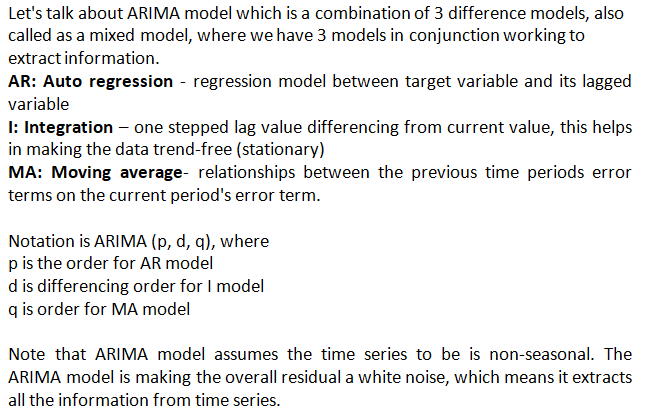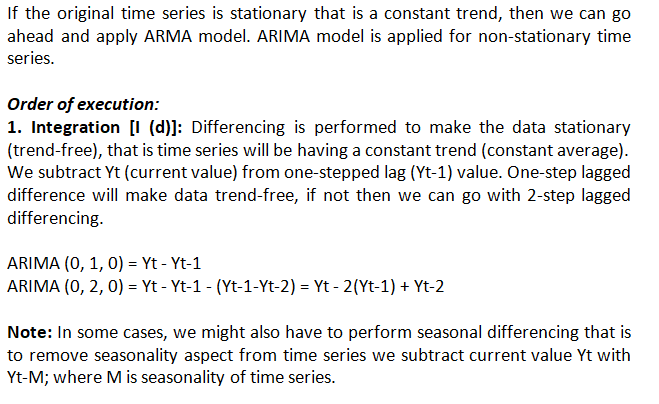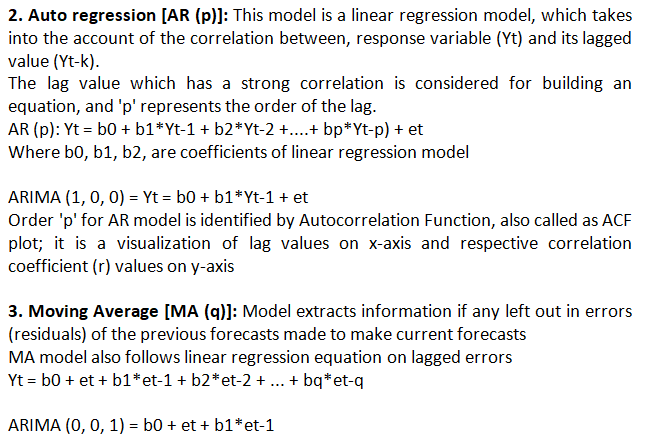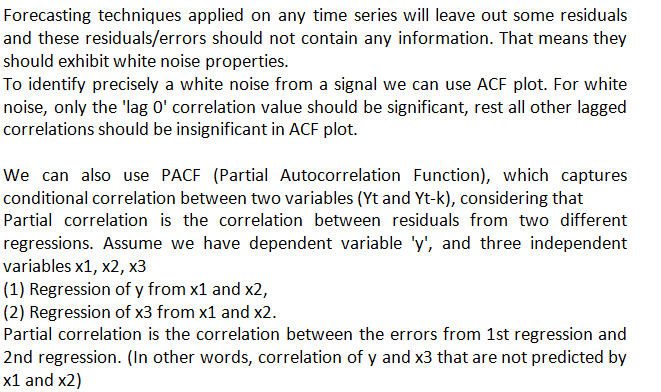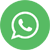Time Series Forecasting Using ARIMA Model

## Time Series Forecasting Using ARIMA Model

Fortune tellers, Horoscopes and Soothsayers are always valued very high, as we are worried about our future and obsessed to know it upfront.

Time series modeling/forecasting are scientific ways to predict the future. We try to estimate a dependent variable based on how it changed over time.

Time series data is collected in sequence and at equal intervals of time. Other types of data, wherein we ignore the effect of time, are called Cross-sectional data.

The conventional data models look into the relationship/effect of independent variables to predict a dependent variable.

In time series data we look at the effect of time on the dependent variable, means time is the independent variable.

We can use a simple linear regression model to predict the dependent variable using time. These type of models which deal with dependent and independent variables are called "Model-based forecasting models".

In few scenarios, we might not look into other independent variables effect on target variable. Models, where dependent and independent variables are same are called as "Data-Driven forecasting models".